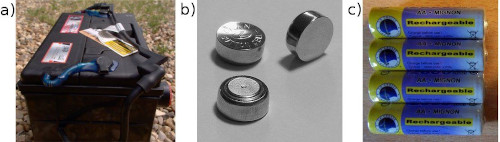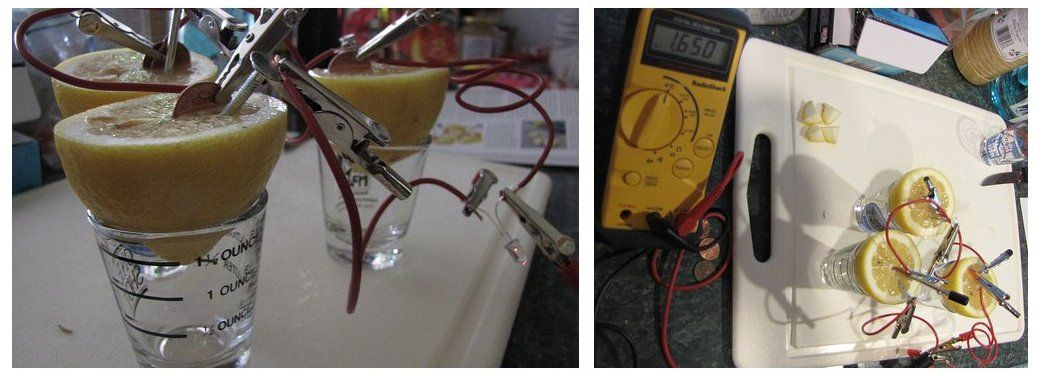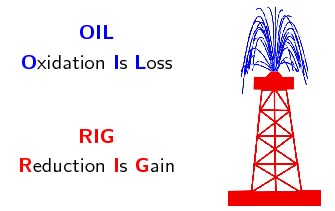Home Practice
For learners and parents For teachers and schools
Textbooks
Full catalogue
Pricing SupportLog in

We think you are located in United States. Is this correct?

# Chapter 13: Electrochemical reactions

This section is allocated $$\text{8}$$ hours in CAPS and covers electrochemical reactions, the galvanic cell, the electrolytic cell, and applications of electrochemistry. The first step should be to revise redox reactions, oxidation numbers, and oxidation and reduction before continuing with the chapter. Note that the sections are not ordered in this way in the CAPS document, but learners are more likely to struggle if you do not revise these concepts first. A good understanding of these topics is essential.

The chapter ends with applications of electrochemisty in industry, this is a good opportunity to make sure the learners understand the principles, but it is not necessary for them to learn the reactions by heart or study the industry itself.

The following topics are covered in this chapter.

• Revision of oxidation, reduction and redox reactions

It is important to revise the concepts of oxidation number, oxidation and reduction reactions, as well as how to write and balance redox reactions and half-reactions. An understanding of these concepts is very important in every aspect of this chapter.

• Galvanic and electrolytic cells

This section introduces the concept of an electrochemical reaction, it may be worth a brief revision of the zinc-copper experiment performed in Grade 11. Two types of electrochemical cell are covered: galvanic cells and electrolytic cells. Galvanic cells convert chemical potential energy to electrical potential energy, while electrolytic cells convert electrical potential energy to chemical potential energy. Learners should be able to draw and label a simple diagram of both of these types of cells and write the standard cell notation for the cell.

• Processes in electrochemical cells

This section is a more indepth look at the oxidation and reduction reactions taking place at the anode and cathode in electrochemical cells. Learners should be able to predict whether an electrode is the anode or cathode depending on the half-reaction taking place there. They must also know which half-reaction will take place at the anode and which will take place at the cathode.

• Effects of current and potential on rate and equilibrium

Learners should understand that when a cell is flat the reactions inside are in equilibrium and the concentration of the reactants and products are constant. There is no potential difference and so no current can flow. The more current there is, the faster the electrons are flowing and the higher the rate of the reaction must be. None of the cells they deal with in this chapter are in equilibrium, because then there would be nothing to measure.

• Standard electrode potentials

A standard hydrogen electrode is used to help determine the EMF of cells. The learners should understand that the electrode potentials in the table of standard electrode potentials are relative electrode potentials, and allow us to compare different metals without having to set up a new reaction each time. They should realise that the table provided is for the reduction half-reactions and that the oxidation half-reaction table would have the reactions reversed and the $$E^{\circ}$$ V value with the opposite sign. They need to be able to use the table to:

• determine which metal is more likely to be reduced or oxidised when comparing two metals

• and determine whether a reaction is spontaneous or not.

• Applications of electrochemistry

The applications covered in this section are electroplating, the chloralkali industry, and the extraction of aluminium. The learners should understand the processes involved, but do not need to memorise the exact reactions, and need not study the industries themselves.

There are several experiments in this chapter. The learners will be using dangerous chemicals and should be properly instructed on the correct use of safety equipment, including safety goggles, gloves and protective clothing. They should also be reminded not to sniff any chemicals as the fumes can be dangerous as well. More information on laboratory procedures as well as safety precautions is provided in Chapter 1 (Science skills).

The informal experiment is the electrolysis of sodium iodide and water. It is a very effective experiment, and should be easy to perform. The learners will need to be careful as they handle concentrated sulfuric acid. It is advisable to test that current will run through the pencils before the experiment, in case the pencil lead is broken inside. If you have access to graphite rods these can be used instead.

We use batteries throughout our day-to-day lives. Cell phones use lithium-ion batteries, cars use lead-acid batteries (Figure 13.1 (left)), while silver-oxide batteries (Figure 13.1 (top right)) are used in watches. Some batteries are rechargeable (Figure 13.1 (bottom right)), while others cannot be recharged and have to be thrown away.Figure 13.1: a) A car battery, b) rechargeable AA batteries and c) watch batteries.

A battery consists of multiple electrochemical cells. And within each cell there are electrochemical reactions taking place. This can be seen in the lemon battery experiment shown in Figure 13.2. Each lemon is a cell in the battery, which consists of three lemon cells. The reactions the copper and zinc undergo in the lemons are electrochemical reactions, and a current is produced.Figure 13.2: A current is produced by connecting lemons with zinc and copper metal. The reactions taking place here are electrochemical reactions.

Electrochemical reactions, and electrochemical cells are covered in this chapter. Before going into any more detail however, it is important to revise oxidation and reduction, as well as redox reactions and how to balance them, as these concepts are very important in electrochemistry.

## 13.1 Revision of oxidation and reduction (ESCQX)

temp text

You should remember the terms oxidation and reduction from Grade 11:

• $$\color{blue}{\textbf{Oxidation}}$$ involves a loss of electrons

• $$\color{red}{\textbf{Reduction}}$$ involves a gain of electrons.

An easy way to remember this is:In both oxidation and reduction a transfer of electrons is involved resulting in a change in the oxidation state of the elements.

• An element or compound that loses electrons is $$\color{blue}{\textbf{oxidised}}$$.

e.g. $$\color{blue}{\textbf{Zn(s)} \to {\textbf{Zn}}^{\textbf{2+}} \textbf{(aq) + 2e}^{\textbf{-}}}$$

As it loses electrons it gives them away to another element or compound and the element or compound it gives the electrons to is $$\color{red}{\textbf{reduced}}$$.

This makes the compound or element which loses electrons a $$\color{blue}{\textbf{reducing agent}}$$.

• An element or compound that gains electrons is $$\color{red}{\textbf{reduced}}$$.

e.g. $$\color{red}{\textbf{Cu}^{\textbf{2+}}{\textbf{(aq) + 2e}}^{\textbf{-}} \to \textbf{Cu(s)}}$$

As it gains electrons it takes them away from another element or compound and the element or compound it takes them from is $$\color{blue}{\textbf{oxidised}}$$.

This makes the compound or element which gains electrons an $$\color{red}{\textbf{oxidising agent}}$$.

## Oxidation and reduction

Textbook Exercise 13.1

Define the following terms:

oxidation

Oxidation is the loss of electrons by a molecule, atom or ion.

reduction

Reduction is the gain of electrons by a molecule, atom or ion.

oxidising agent

The molecule, atom or ion that is reduced, or the molecule, atom or ion that causes oxidation.

reducing agent

The molecule, atom or ion that is oxidised, or the molecule, atom or ion that causes reduction.

In each of the following reactions say whether the reactant iron species ($$\text{Fe}$$, $$\text{Fe}^{2+}$$, $$\text{Fe}^{3+}$$) is oxidised or reduced.

$$\text{Fe}(\text{s})$$ $$\to$$ $$\text{Fe}^{2+}(\text{aq}) + 2\text{e}^{-}$$

The oxidation number of $$\text{Fe}$$ in:

• $$\text{Fe}(\text{s})$$ is $$\text{0}$$

• $$\text{Fe}^{2+}(\text{aq})$$ is $$\text{+2}$$

$$\text{Fe}(\text{s})$$ $$\to$$ $$\text{Fe}^{2+}(\text{aq}) + 2\text{e}^{-}$$

$$\text{Fe}$$ loses two electrons to become $$\text{Fe}^{2+}$$. The iron species ($$\text{Fe}(\text{s})$$) is oxidised.

$$\text{Fe}^{3+}(\text{aq}) + \text{e}^{-}$$ $$\to$$ $$\text{Fe}^{2+}(\text{aq})$$

The oxidation number of $$\text{Fe}$$ in:

• $$\text{Fe}^{3+}(\text{aq})$$ is $$\text{+3}$$

• $$\text{Fe}^{2+}(\text{aq})$$ is $$\text{+2}$$

$$\text{Fe}^{3+}(\text{aq}) + \text{e}^{-}$$ $$\to$$ $$\text{Fe}^{2+}(\text{aq})$$

$$\text{Fe}^{3+}$$ gains one electron to become $$\text{Fe}^{2+}$$. The iron species ($$\text{Fe}^{3+}(\text{aq})$$) is reduced.

$$\text{Fe}_{2}\text{O}_{3}(\text{s}) + 3\text{CO}(\text{g})$$ $$\to$$ $$2\text{Fe}(\text{s}) + 3\text{CO}_{2}(\text{g})$$

The oxidation number of $$\text{O}$$ is $$-\text{2}$$. Therefore the oxidation number of $$\text{O}_{3}$$ in $$\text{Fe}_{2}\text{O}_{3}$$ is $$-\text{6}$$.

Therefore the oxidation number of $$\text{Fe}_{2}$$ in $$\text{Fe}_{2}\text{O}_{3}$$ is $$\text{+6}$$.

The oxidation number of $$\text{Fe}$$ in:

• $$\text{Fe}_{2}\text{O}_{3}(\text{s})$$ is $$\text{+3}$$

• $$\text{Fe}(\text{s})$$ is $$\text{0}$$

$$\text{Fe}^{3+}(\text{s}) + 3\text{e}^{-}$$ $$\to$$ $$\text{Fe}(\text{s})$$

$$\text{Fe}^{3+}$$ gains three electrons to become $$\text{Fe}(\text{s})$$. The iron species ($$\text{Fe}^{3+}(\text{s})$$) is reduced.

$$\text{Fe}^{2+}(\text{aq})$$ $$\to$$ $$\text{Fe}^{3+}(\text{aq}) + \text{e}^{-}$$

The oxidation number of $$\text{Fe}$$ in:

• $$\text{Fe}^{2+}(\text{aq})$$ is $$\text{+2}$$

• $$\text{Fe}^{3+}(\text{aq})$$ is $$\text{+3}$$

$$\text{Fe}^{2+}(\text{aq})$$ $$\to$$ $$\text{Fe}^{3+}(\text{aq}) + \text{e}^{-}$$

$$\text{Fe}^{2+}$$ loses an electron to become $$\text{Fe}^{3+}$$. The iron species ($$\text{Fe}^{2+}(\text{aq})$$) is oxidised.

$$\text{Fe}_{2}\text{O}_{3}(\text{s}) + 2\text{Al}(\text{s})$$ $$\to$$ $$\text{Al}_{2}\text{O}_{3}(\text{s}) + 2\text{Fe}(\text{s})$$

The oxidation number of $$\text{O}$$ is $$-\text{2}$$. Therefore the oxidation number of $$\text{O}_{3}$$ in $$\text{Fe}_{2}\text{O}_{3}$$ is $$-\text{6}$$.

Therefore the oxidation number of $$\text{Fe}_{2}$$ in $$\text{Fe}_{2}\text{O}_{3}$$ is $$\text{+6}$$.

The oxidation number of $$\text{Fe}$$ in:

• $$\text{Fe}_{2}\text{O}_{3}(\text{s})$$ is $$\text{+3}$$

• $$\text{Fe}(\text{s})$$ is $$\text{0}$$

$$\text{Fe}^{3+}(\text{s}) + 3\text{e}^{-}$$ $$\to$$ $$\text{Fe}(\text{s})$$

$$\text{Fe}^{3+}$$ gains three electrons to become $$\text{Fe}(\text{s})$$. The iron species ($$\text{Fe}^{3+}(\text{aq})$$) is reduced.